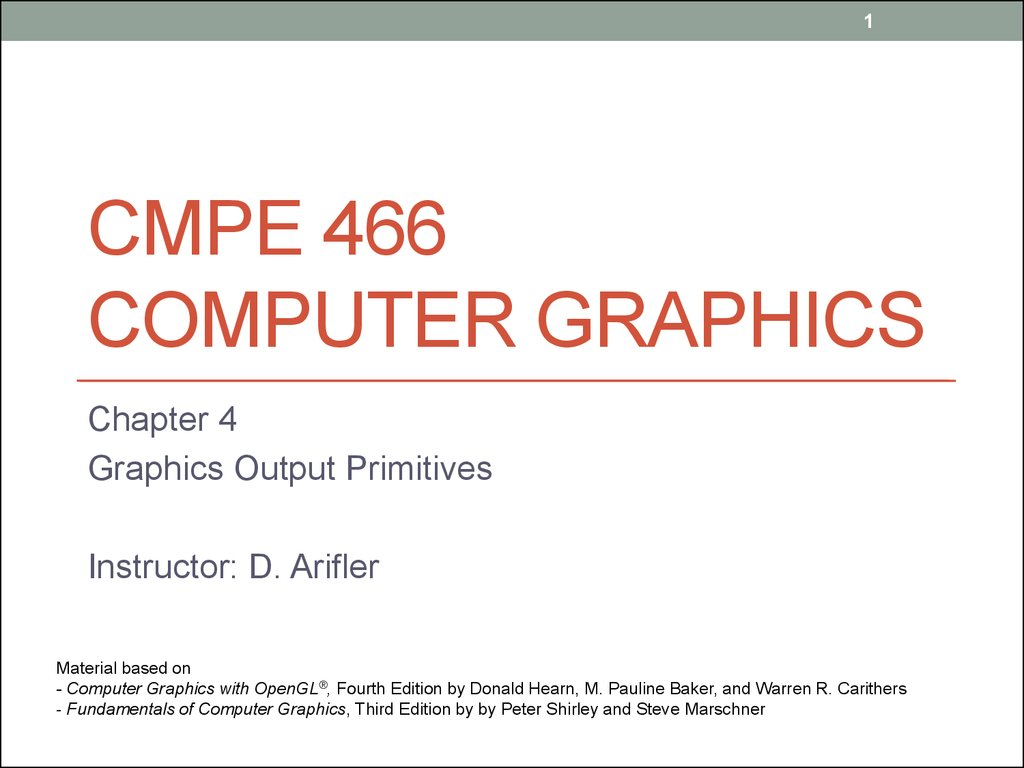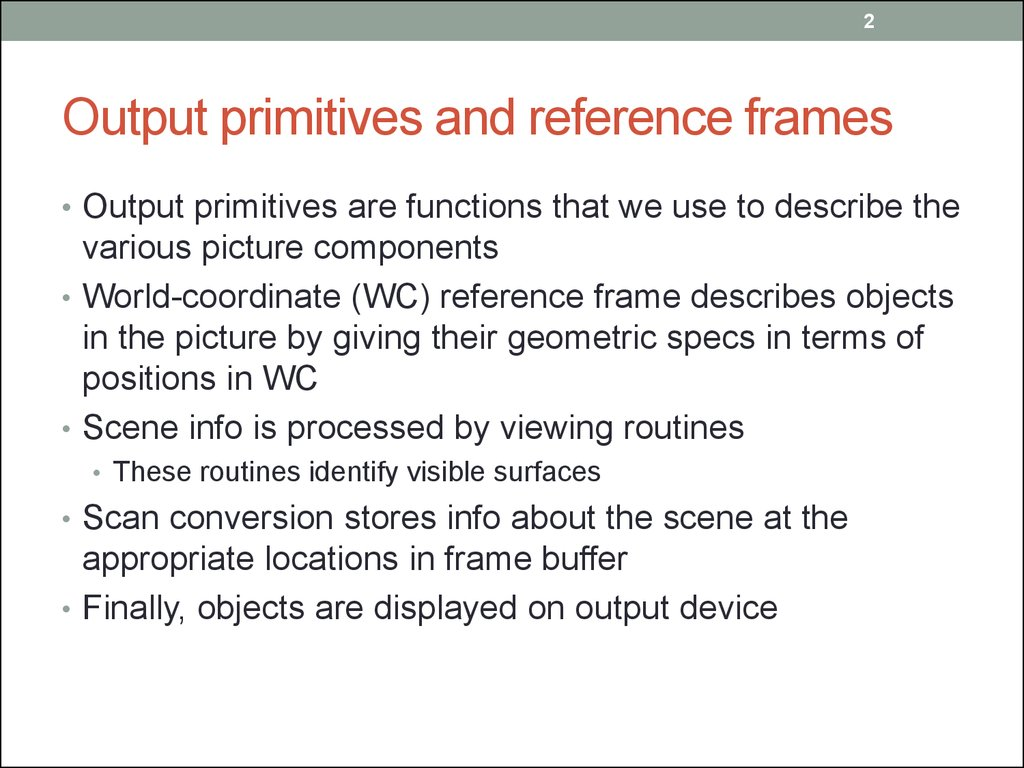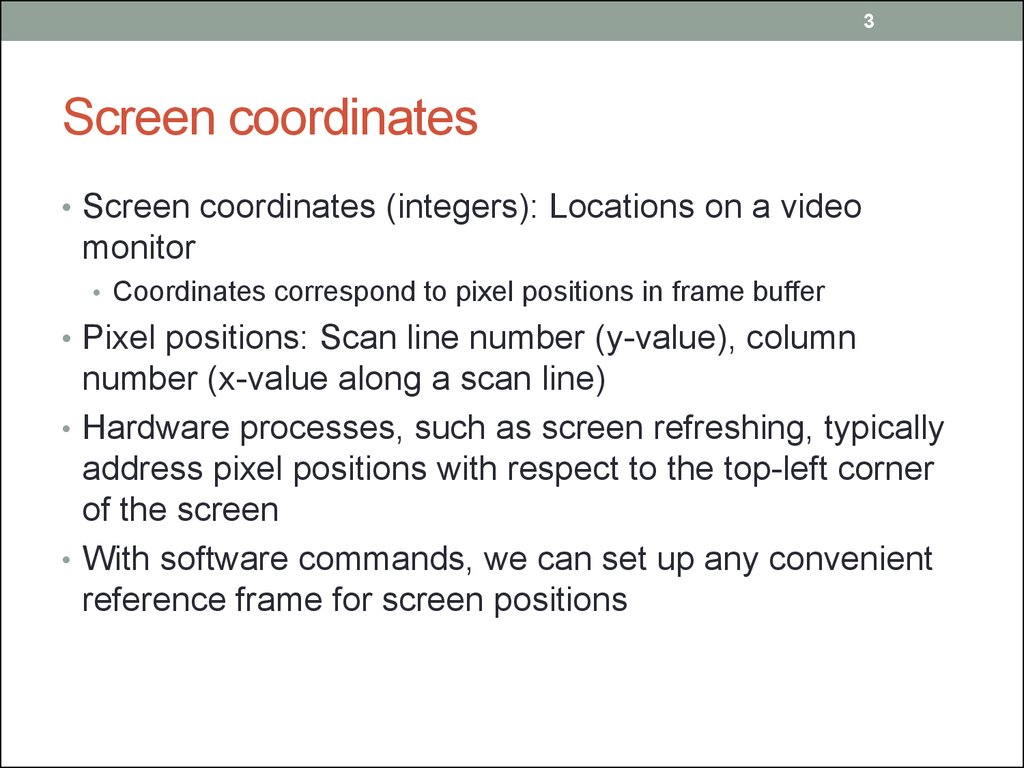# Cmpe 466 computer graphics. Graphics output primitives. (Chapter 4)

## 1. CMPE 466 COMPUTER GRAPHICS

1
CMPE 466
COMPUTER GRAPHICS
Chapter 4
Graphics Output Primitives
Instructor: D. Arifler
Material based on
- Computer Graphics with OpenGL®, Fourth Edition by Donald Hearn, M. Pauline Baker, and Warren R. Carithers
- Fundamentals of Computer Graphics, Third Edition by by Peter Shirley and Steve Marschner

## 2. Output primitives and reference frames

2
Output primitives and reference frames
• Output primitives are functions that we use to describe the
various picture components
• World-coordinate (WC) reference frame describes objects
in the picture by giving their geometric specs in terms of
positions in WC
• Scene info is processed by viewing routines
• These routines identify visible surfaces
• Scan conversion stores info about the scene at the
appropriate locations in frame buffer
• Finally, objects are displayed on output device

## 3. Screen coordinates

3
Screen coordinates
• Screen coordinates (integers): Locations on a video
monitor
• Coordinates correspond to pixel positions in frame buffer
• Pixel positions: Scan line number (y-value), column
number (x-value along a scan line)
• Hardware processes, such as screen refreshing, typically
address pixel positions with respect to the top-left corner
of the screen
• With software commands, we can set up any convenient
reference frame for screen positions

## 4. Specifying a 2D WC reference frame

4
Specifying a 2D WC reference frame
Figure 4-2 World-coordinate limits for a display window, as specified in the
glOrtho2D function.
glMatrixMode (GL_PROJECTION);
gluOrtho2D (xmin, xmax, ymin, ymax);

## 5. OpenGL point functions

5
OpenGL point functions
Figure 4-3 Display of three point positions generated with glBegin
(GL_POINTS).
glBegin (GL_POINTS);
glVertex2i (50, 100);
glVertex2i (75, 150);
glVertex2i (100, 200);
glEnd ( );

## 6. OpenGL point functions: alternative code

6
OpenGL point functions: alternative code
Figure 4-3 Display of three point positions generated with glBegin
(GL_POINTS).
int point1 [ ] = {50, 100};
int point2 [ ] = {75, 150};
int point3 [ ] = {100, 200};

glBegin (GL_POINTS);
glVertex2iv (point1);
glVertex2iv (point2);
glVertex2iv (point3);
glEnd ( );

## 7. More on point functions

7
More on point functions
• Specifying positions in 3D using floating-point coordinates
glBegin (GL_POINTS);
glVertex3f (-78.05, 909.72, 14.60);
glVertex3f (261.91, -5200.67, 188.33);
glEnd ( );
• Using class or struct to specify point positions
class wcPt2D {
public:
GLfloat x, y;
};
wcPt2D pointPos;
pointPos.x = 120.75;
pointPos.y = 45.30;
glBegin (GL_POINTS);
glVertex2f (pointPos.x, pointPos.y);
glEnd ( );

## 8. OpenGL line functions

8
OpenGL line functions
Figure 4-4 Line segments that can be displayed in OpenGL using a list of five endpoint coordinates. (a) An unconnected
set of lines generated with the primitive line constant GL_LINES. (b) A polyline generated with GL_LINE_STRIP. (c) A
closed polyline generated with GL_LINE_LOOP.
glBegin (GL_LINES);
glVertex2iv (p1);
glVertex2iv (p2);
glVertex2iv (p3);
glVertex2iv (p4);
glVertex2iv (p5);
glEnd ( );
glBegin (GL_LINE_STRIP); glBegin (GL_LINE_LOOP);
glVertex2iv (p1);
glVertex2iv (p1);
glVertex2iv (p2);
glVertex2iv (p2);
glVertex2iv (p3);
glVertex2iv (p3);
glVertex2iv (p4);
glVertex2iv (p4);
glVertex2iv (p5);
glVertex2iv (p5);
glEnd ( );
glEnd ( );

## 9. Polygon Fill Areas

9
Polygon Fill Areas
Figure 4-8 A convex polygon (a), and a concave polygon (b).
Concave polygons may present
problems when implementing fill
algorithms

## 10. Identifying concave polygons

10
Identifying concave polygons
Figure 4-9 Identifying a concave polygon by calculating cross-products of
successive pairs of edge vectors.
For concave polygons, some cross-products are positive, some are negative

## 11. Splitting concave polygons: vector method

11
Splitting concave polygons: vector method
• Form the edge vectors Ek=Vk+1 - Vk
• Calculate the cross-products of successive edge vectors
in a counter-clockwise manner
• Use the cross-product test to identify concave polygons
• If any cross-product has a negative z-component, the
polygon is concave and we can split it along the line of the
first edge vector in the cross-product pair

## 12. Splitting example

12
Splitting example
Figure 4-10 Splitting a concave
polygon using the vector method.

## 13. Splitting polygons

13
Splitting polygons

## 14. Splitting a convex polygon into a set of triangles

14
Splitting a convex polygon into a set of triangles
• Triangles make several important processing routines
simple
Define any sequence of three consecutive vertices to be a
new polygon (triangle)
Delete the middle triangle vertex from the original vertex
list
Apply the same procedure to the modified list to strip off
another triangle
Continue until the original polygon is reduced to just three
vertices (the last triangle)

## 15. Inside-outside tests

15
Inside-outside tests
• Odd-even rule
• Draw a line from any position P to a distant point outside
the coordinate extents of the closed polyline
• Count the number of line segment crossings along this
line
• If odd, P is an interior point
• Otherwise, P is an exterior point
• Note that the line must not intersect any line segment
end-points

## 16. Nonzero winding-number rule

16
Nonzero winding-number rule
• Object edges and the line must be vectors
• Count the number of times that the boundary of an object
“winds” around a particular point in the counter-clockwise
direction
• Interior points are those that have nonzero value for the winding
number
• Draw a line from P to a distant point
• As we move along the line from P, count the number of object
line segments that cross the reference line in each direction
• Add 1 to the winding number every time we intersect a segment that
crosses the line from right to left
• Subtract 1 if crossed from left to right
• If the final value of winding number is nonzero, P is an interior point;
otherwise, it is an exterior point
• For simple objects, both rules give the same results

## 17. Inside-outside test examples

17
Inside-outside test examples
Figure 4-12 Identifying interior and exterior regions of a closed polyline that contains self-intersecting
segments.

## 18. Variations of nonzero winding-number rule

18
Variations of nonzero winding-number rule
Figure 4-13 A fill area defined as a region that has a positive value for the winding number. This fill
area is the union of two regions, each with a counterclockwise border direction.

## 19. Variations of nonzero winding-number rule

19
Variations of nonzero winding-number rule
Figure 4-14 A fill area defined as a region with a winding number greater than 1. This fill area is
the intersection of two regions, each with a counterclockwise border direction.

## 20. Variations of nonzero winding-number rule

20
Variations of nonzero winding-number rule
Figure 4-15 A fill area defined as a region with a positive value for the winding number. This fill area is the
difference, A − B, of two regions, where region A has a positive border direction (counterclockwise) and
region B has a negative border direction (clockwise).

## 21. Polygon tables

21
Polygon tables
Figure 4-16 Geometric data-table representation for two adjacent polygon
surface facets, formed with six edges and five vertices.
A surface shape can
be defined as a
mesh of polygon
patches. Geometric
data for objects can
be arranged in 3 lists

## 22. Plane equations

22
Plane equations
• Often, information about spatial orientation of surface
components is needed
• This info is obtained from the equations that describe
polygon surfaces
• Each polygon in a scene is contained within a plane of
infinite extent
• The general equation of a plane is
where (x, y, z) is any point on the plane

## 23. Solutions to plane equations

23
Solutions to plane equations
• We obtain A, B, C, and D by solving a set of three plane
equations
• Select 3 successive convex polygon vertices in a counter-clockwise
manner and solve the following set of simultaneous linear plane
equations for A/D, B/D, and C/D
• Solution to this set of equations can be obtained using Cramer’s
rule

24
Solutions
• Then

## 25. Front and back polygon faces

25
Front and back polygon faces
• Faces can be determined by the sign of Ax+By+Cz+D
Figure 4-19 The normal vector N for a plane described with the equation Ax + By +Cz + D
= 0 is perpendicular to the plane and has Cartesian components (A, B, C) .

## 26. OpenGL polygon fill-area functions

26
OpenGL polygon fill-area functions
Figure 4-22 Displaying polygon fill areas using a list of six vertex positions. (a) A single convex
polygon fill area generated with the primitive constant GL_POLYGON. (b) Two unconnected
triangles generated with GL_ TRIANGLES. (c) Four connected triangles generated with
GL_TRIANGLE_STRIP. (d) Four connected triangles generated with GL_TRIANGLE_FAN.

## 27. Fill-area examples

27
Fill-area examples

28
Figure 4-23 Displaying quadrilateral fill areas
using a list of eight vertex positions. (a) Two

## 29. A complex scene might require hundreds or thousands of OpenGL calls!

29
A complex scene might require hundreds or
thousands of OpenGL calls!
Figure 4-24 A cube with an
edge length of 1.

## 30. Using a vertex array

30
Using a vertex array
Figure 4-25 Subscript values for array pt
corresponding to the vertex coordinates
for the cube shown in Figure 4-24.
{
}

## 31. Display lists

31
Display lists
const double TWO_PI = 6.2831853;
GLuint regHex;
GLdouble theta;
GLint x, y, k;
/* Set up a display list for a regular
hexagon.
* Vertices for the hexagon are six
equally spaced
* points around the circumference of
a circle.
*/
regHex = glGenLists (1); // Get an
identifier for the display list.
glNewList (regHex, GL_COMPILE);
glBegin (GL_POLYGON);
for (k = 0; k < 6; k++) {
theta = TWO_PI * k / 6.0;
x = 200 + 150 * cos (theta);
y = 200 + 150 * sin (theta);
glVertex2i (x, y);
}
glEnd ( );
glEndList ( );
glCallList (regHex);

## 32. OpenGL display-window reshape function

32
OpenGL display-window reshape function
• glutReshapeFunc (winReshapeFcn);
• Activated whenever display-window size is changed